Courses

# Olympiad Test : Geometrical Shapes

## 20 Questions MCQ Test Science Olympiad for Class 5 | Olympiad Test : Geometrical Shapes

Description
This mock test of Olympiad Test : Geometrical Shapes for Class 5 helps you for every Class 5 entrance exam. This contains 20 Multiple Choice Questions for Class 5 Olympiad Test : Geometrical Shapes (mcq) to study with solutions a complete question bank. The solved questions answers in this Olympiad Test : Geometrical Shapes quiz give you a good mix of easy questions and tough questions. Class 5 students definitely take this Olympiad Test : Geometrical Shapes exercise for a better result in the exam. You can find other Olympiad Test : Geometrical Shapes extra questions, long questions & short questions for Class 5 on EduRev as well by searching above.
QUESTION: 1

Solution:
QUESTION: 2

Solution:
QUESTION: 3

### ​How many triangles does the following figure have?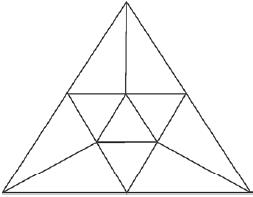Solution:
QUESTION: 4

How many triangles and squares does the following figure have?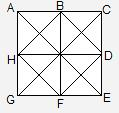Solution:
QUESTION: 5

How many straight lines does the following figure have?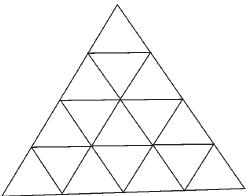Solution:
QUESTION: 6

In each of the following questions a part of the figure is missing. Find out the light figure from the given alternatives (A), (B), (C) and (D), which will complete the figure .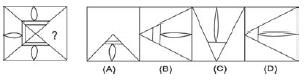Solution:
QUESTION: 7

In each of the following questions a part of the figure is missing. Find out the light figure from the given alternatives (A), (B), (C) and (D), which will complete the figure .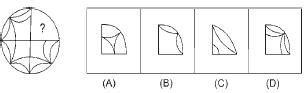Solution:
QUESTION: 8

In each of the following questions a part of the figure is missing. Find out the light figure from the given alternatives (A), (B), (C) and (D), which will complete the figure .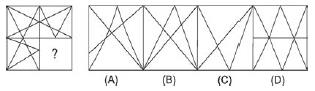Solution:
QUESTION: 9

In each of the following questions a part of the figure is missing. Find out the light figure from the given alternatives (A), (B), (C) and (D), which will complete the figure .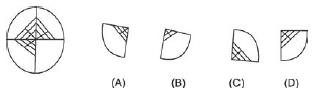Solution:
QUESTION: 10

In each of the following questions a part of the figure is missing. Find out the light figure from the given alternatives (A), (B), (C) and (D), which will complete the figure .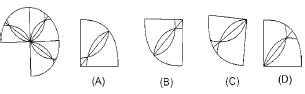Solution:
QUESTION: 11

In each of the following questions a part of the figure is missing. Find out the light figure from the given alternatives (A), (B), (C) and (D), which will complete the figure .
Q. Which of the following dice is identical to the unfolded figure shown below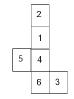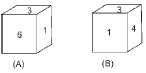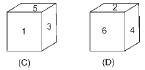Solution:
QUESTION: 12

In each of the following questions a part of the figure is missing. Find out the light figure from the given alternatives (A), (B), (C) and (D), which will complete the figure .
Q. How many squares are in following figure?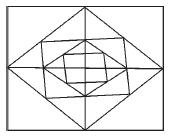Solution:
QUESTION: 13

Select a figure from the given alternatives which when placed in the blank space of figure (X) would complete the pattern.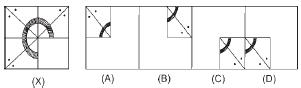Solution:
QUESTION: 14

Select a figure from the given alternatives which when placed in the blank space of figure (X) would complete the pattern.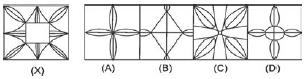Solution:
QUESTION: 15

Select a figure from the given alternatives which when placed in the blank space of figure (X) would complete the pattern.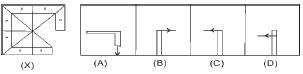Solution:
QUESTION: 16

Select a figure from the given alternatives which when placed in the blank space of figure (X) would complete the pattern.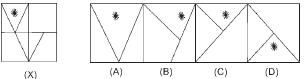Solution:
QUESTION: 17

Select a figure from the given alternatives which when placed in the blank space of figure (X) would complete the pattern.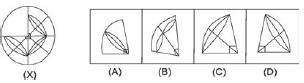Solution:
QUESTION: 18

Select a figure from the given alternatives which when placed in the blank space of figure (X) would complete the pattern.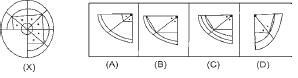Solution:
QUESTION: 19

Select a figure from the given alternatives which when placed in the blank space of figure (X) would complete the pattern.
Q. No. of straight line in the following figure are: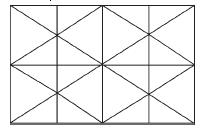Solution:
QUESTION: 20

Select a figure from the given alternatives which when placed in the blank space of figure (X) would complete the pattern.
Q. No. of parallelograms in the following figure are: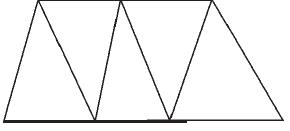Solution: